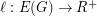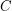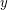# Geodesic cycles and Tutte's Theorem

 Importance: Medium ✭✭
 Author(s): Georgakopoulos, Agelos Sprüssel, Philipp
 Subject: Graph Theory » Basic Graph Theory » » Cycles
 Keywords: cycle space geodesic cycles peripheral cycles
 Posted by: Agelos on: August 4th, 2007
Problem   Ifis a-connected finite graph, is there an assignment of lengthsto the edges of, such that every-geodesic cycle is peripheral?

A cycleis-geodesic if for every two verticesonthere is no-~path inshorter, with respect to, than both-~arcs on.

It is not hard to prove [GS] that for every finite graphand every assignment of edge lengthsthe-geodesic cycles ofgenerate its cycle space. Thus, a positive answer to the problem would imply a new proof of Tutte's classical theorem [T] that the peripheral cycles of a-connected finite graph generate its cycle space.

## Bibliography

*[GS] Angelos Georgakopoulos, Philipp Sprüssel: Geodesic topological cycles in locally finite graphs. Preprint 2007.

[T] W.T. Tutte, How to draw a graph. Proc. London Math. Soc. 13 (1963), 743–768.

* indicates original appearance(s) of problem.